# Interpreting summary of peers and projected values in VRS-DEA

By Avishek Majumder & Priya Chetty on November 4, 2017

This is the continued article of the interpretations for variable returns to scale (VRS) from the last article. However, this article is about the summary of peers and the rest of the analysis conducted for VRS Data envelopment analysis (DEA).

## Summary of peers

The value and the reference of the organizations that are to be adopted are presented by the summary of peers. Whereas, the percentage variation indicates the peer weights in order to adopt the trend of the reference organization to become efficient. The peer count indicates the number of times an efficient organization acts as a reference for the inefficient organizations.

### Interpretations

Banks 1, 2, 3, 4, 5 and 6 are to refer Banks 7, 8, 9 and 10 to become effective for input oriented VRSDEA. Thus, all the public banks which are inefficient can follow the efficiency trend of private banks 7, 8, 9 and 10. Similarly, in case of output-oriented DEA, Banks 1, 2, 3, 4, 5 and 6 can refer Total profits and Loans of Bank 7, 8, 9 and 10 to become efficient.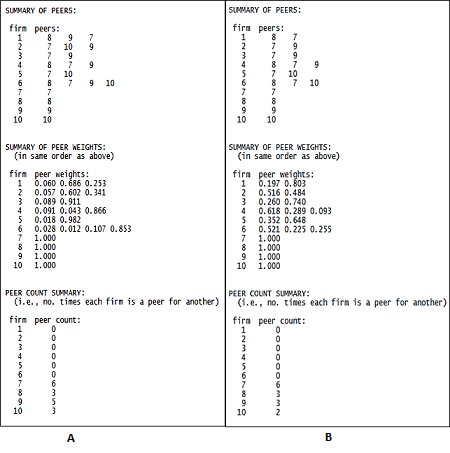Summary of Peers (A) Input oriented VRS DEA (B) Output oriented VRS–DEA

## Summary of targets

To become efficient, a sample financial model represents the summary of targets. 33844.140 and 144712.454 are the Bank 1’s summary of targets for both input oriented VRSDEA with respect to Total Capital and Deposit. For output oriented analysis with respect to efficient returns to scale, Total Capitals and Deposits must be 101187.702 and 365450.361. Thus, Bank 1 would achieve Loans of 101187.702 and Total profits of 365450.360.

### Interpretations

However, one distinctive difference that can be observed for Bank 2 as shown in image A below, where values for Profits remain constant while the other variables such as Total Capitals, Deposits and Loans variation is mainly because this is a VRS DEA and can be compared to as the major difference between CRS DEA model (Cooper, Seiford, & Zhu, 2011). The values changed, mainly because Bank 2 (Bank) has increasing returns to scale (Benicio & De Mello, 2015). Thus, by either increasing or decreasing the values for Total Capitals (10220.479), Deposits (36597.028) and Loans (294159.471) for the given targets, the bank can become efficient. However, similar interpretations are possible for image B too.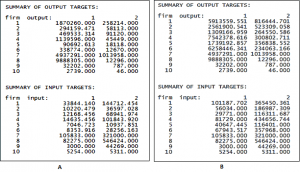A) Summary for Input oriented VRS DEA B) Summary for Output oriented VRS DEA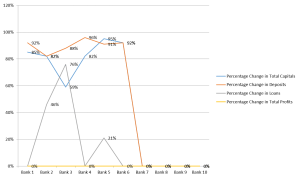Graph showing percentage change of Total capitals, Deposits, Loans and Total Profits for the banks to become efficient (Input oriented VRS–DEA)

The graph shows that for Bank 1 (SBI Bank), to become effective, it has to change its finances up to 92% for Deposits and 85% for Total Capitals. However, no change required for Bank 1 (SBI) with respect to Loans or Total Profits.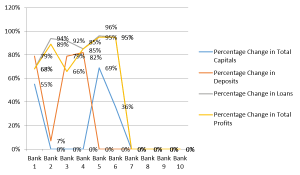Graph showing percentage change of Total capitals, Deposits, Loans and Total Profits for the banks to become efficient (Output oriented VRS–DEA)

The inefficient Bank 2 (Allahabad bank) has to change its total capital by 55%, deposits by 5%, loans by 68% and profits by 68%, in order to become efficient. Thus, changing the finances by this percentage, bank 2 can become efficient. While banks showing 0% change means that they do not have to change that specific value to become efficient.

## Radial movement, slack movement, and projected value of VRS-DEA

As mentioned in the previous articles, the radial movement shows proportional change, however, since VRSDEA frontier does not work on the basis of proportional change and rather works on the laws of returns IRS and DRS, so this is an exception where the proportional change occurs due to the evaluation of scale efficiency measurement (Podinovski, 2004). Thus, here proportional change occurs so as to provide information on scale efficiency and thus increasing or decreasing returns to scale (Coelli, 2008).

The models indicate a projected value for input oriented CRS whereby the output values remain constant, and vice versa in case of output oriented. Unlike CRS, in VRS model the variables change is based on the increasing or decreasing returns to scale (Tsai & Mar Molinero, 2002). The image A below, for input oriented VRS DEA, Bank 2 has all the projected values changed. However, the interpretations of the projected values remain the same as in CRS DEA.

### Interpretations

For Bank 2, if the Total Capital and Deposits are 10220.479 and 36597.028 respectively, the variable increase in returns to scale will be 294159.471 for Loans and 58113.000 for Total profits. Thus, Bank 1 (SBI) can decrease total capitals and deposits (radial movement) to achieve the projected variables in order to make the bank efficient. Applying these projected values the Bank 2 can become efficient. The projected values for Total capitals and Deposits are adopted to achieve efficient variable returns to scale.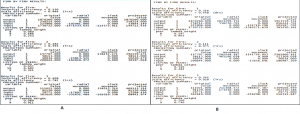Projected value, Radial movement and Slack Movement A) Summary for Input oriented VRS DEA B) Summary for Output oriented VRS DEA